# Paper Review: Self-Normalizing Neural Networks

One of the main problems of neural networks is to tame layer activations so that one is able to obtain stable gradients to learn faster without any confining factor. Batch Normalization shows us that keeping values with mean 0 and variance 1 seems to work things. However, albeit indisputable effectiveness of BN, it adds more layers and computations to your model that you'd not like to have in the best case.

ELU (Exponential Linear Unit) is a activation function aiming to tame neural networks on the fly by a slight modification of activation function. It keeps the positive values as it is and exponentially skew negative values.

ELU does its job good enough, if you like to evade the cost of Bath Normalization, however its effectiveness does not rely on a theoretical proof beside empirical satisfaction. And finding a good $\alpha$ is just a guess.

Self-Normalizing Neural Networks takes things to next level. In short, it describes a new activation function SELU (Scaled Exponential Linear Units), a new initialization scheme and a new dropout variant as a repercussion,

The main topic here is to keep network activation in a certain basin defined by a mean and a variance values. These can be any values of your choice but for the paper it is mean 0 and variance 1 (similar to notion of Batch Normalization). The question afterward is to modifying ELU function by some scaling factors to keep the activations with that mean and variance on the fly. They find these scaling values by a long theoretical justification. Stating that, scaling factors of ELU are supposed to be defined as such any passing value of ELU should be contracted to define mean and variance.  (This is just verbal definition by no means complete. Please refer to paper to be more into theory side. )Above, the scaling factors are shown as $\alpha$ and $\lambda$.  After long run of computations these values appears to be 1.6732632423543772848170429916717 and 1.0507009873554804934193349852946 relatively. Nevertheless, do not forget that these scaling factors are targeting specifically mean 0 and variance 1.  Any change preludes to change these values as well.

Initialization is also another important part of the whole method. The aim here is to start with the right values. They suggest to sample weights from a Gaussian distribution with mean 0 and variance $1/n$ where n is number of weights.

It is known with a well credence that Dropout does not play well with Batch Normalization since it smarting network activations in a purely random manner. This method seems even more brittle to dropout effect. As a cure, they propose Alpha Dropout. It randomly sets inputs to saturatied negative value of SELU which is $-\alpha\lambda$. Then an affine transformation is applied to it with $a$ and $b$ values computed relative to dropout rate, targeted mean and variance.It randomizes network without degrading network properties.

In a practical point of view, SELU seems promising by reducing the computation time relative to RELU+BN for normalizing the network. In the paper they does not provide any vision based baseline such a MNIST, CIFAR and they only pounce on Fully-Connected models. I am still curios to see its performance vis-a-vis on these benchmarks agains Bath Normalization. I plan to give it a shoot in near future.

One tickle in my mind after reading the paper is the obsession of mean 0 and variance 1 for not only this paper but also the other normalization techniques. In deed, these values are just relative so why 0 and 1 but not 0 and 4. If you have a answer to this please ping me below.

# Paper Notes: The Shattered Gradients Problem ...

The whole heading of the paper is "The Shattered Gradients Problem: If resnets are the answer, then what is the question?". It is really interesting work with all its findings about gradient dynamics of neural networks. It also examines Batch Normalization (BN) and Residual Networks (Resnet) under this problem.

The problem, dubbed "Shattered Gradients", described as gradient feedbacks resembling random noise for nearby data points. White noise gradients (random value around 0 with some unknown variance) are not useful for training and they stall the network. What we expect to see is Brownian noise (next value is obtained with a small change on the last value) from a working model. Deep neural networks are more prone to white noise gradients. However, latest advances like BN and Resnet are described to be more resilient to random gradients even in deep networks.White noise gradients undermines the effectiveness of networks because they violates gradient based learning methods which expects similar gradient feedbacks for data points close by in the vector space. Once you have white noise gradient for such close points, the model is not able to capture data manifold through these learning algorithms. Brownian updates yields more correlation on updates and this preludes effective learning.

For normal networks, they give a empirical evidence that the correlation of network updates decreases with the order $(/2^L$ where L is number of layers. Decreasing correlation means more white noise gradient feedbacks.

One important reason of white noise feedbacks is to be co-activations of network units. From a working model, we expect to have units receptive to different structures in the given data. Therefore, for each different instance, different subset of units should be active for effective information flux. They observe that as activation goes through layers, co-activation rate goes higher. BN layers prevents this by keeping the co-activation rate 1/4 (1/4 units are active per layer).Beside the co-activation rate, how dispersed units activation is another important question. Thus, similar instances need to activate similar subset of units and activation should be distributes to other subsets as we change the data structure. This stage is where the skip-connections get into the play. Their observation is skip-connections improve networks in that respect. This can be observed at below figure.The effectiveness of skip-connections increases with Beta scaling introduced by InceptionV4 architecture. It  is scaling residual connections by a constant value before summing up with the current layer activation.

#### A small discussion

This is a very intriguing paper to me as being one of the scarse works investigating network dynamics instead of blind updates on architectures for racing accuracy values.

Resnet is known to be train hundreds of layers which was not possible before. Now, with this work, we have another scientific argument explaining its effectiveness. I also like to point Veit et al. (2016) demystifying Resnet as an ensemble of many shallow networks. When we combine both of these papers, it makes total sense to me how Resnets are useful for training very deep networks. If shattered gradient effect, as stated here,  increasing with number of layers with the order 2^L then it is impossible to train hundred layers with an ad-hoc network. Corollary, since Resnet behaves like a ensemble of shallow networks this effects is rehabilitated. We are able to see it empirically in this paper and it is complimentary in that sense.

Note: This hastily written paper note might include any kind of error. Please let me know if you find one. Best 🙂

# My Notes - Weight Normalization

Deep Learning is defined as (Goodfellow et al., 2016) a sub-field of machine learning consists in learning models that are wholly or partially specified by a class of flexible differentiable functions.
In this study there are three main methods which are Weight Normalization, a new data depended initialization method and Mean Only Batch Normalization.
Weight normalization id formalized as below. Weight values w are decoupled by their norms  g and the direction v / ||v||. In this way they propose that SGD gives faster convergence.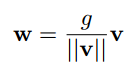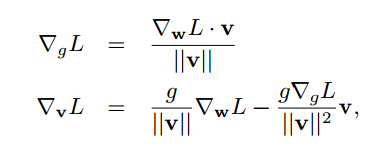They compare Weight Normalization with Batch Normalization. The main disadvantage they posit that BN has stochasticity due to varying data batches and one additional difference is that WN has lower computational burden compared to BN.
the second perk is data depended initialization of the network. They first give a initial minibatch to network and compute mean activation and std per layer. Then given the initial weight values sampled from mean 0 and std 0.05, they set g = 1 / std and b = - mean / std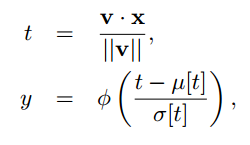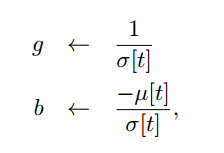One downside is that since this scheme is batch depended, it might suffer for the forthcoming batches with possible different data statistics. However, they say that this scheme works well in practice.
The  third perk is Mean Only Batch Normalization.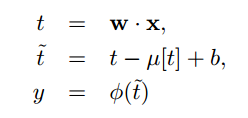This is a lighter operation due to the avoidance of variance normalization. We might easily skip variance normalization because of the initialization scheme already applied it. One another upside is that avodiance of variance normalization provides less distracted gradient feedbacks and therefore better learning.
At the experiments side, they note that batch normalization is 16% slower than weight normalization whereas BN yields better progress especially for initial iterations.  As a final remark they note 7.31% CIFAR-10 performance which is the state of art up to my knowledge (not better then my best network :)) in terms of published works. they also experiment with different architectures like RNNs , reinforcement learning and others but please refer to the paper for more.